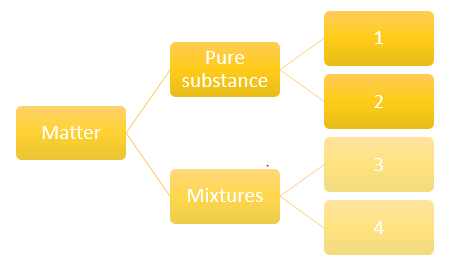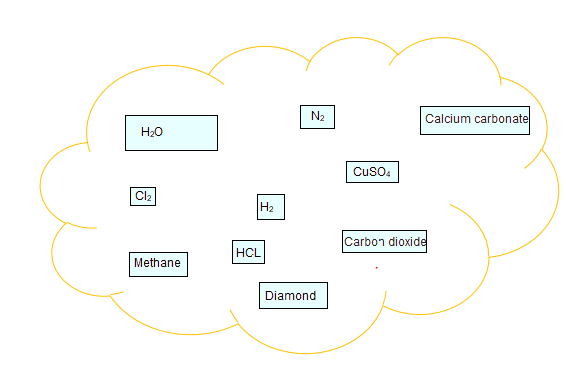# Class 9 Science CBSE Important questions Is Matter around us pure

Given below are the Class 9 Science CBSE Important questions Is Matter around us pure
Hope you like them and do not forget to like , social share and comment at the end of the page.

Question 1. State any one difference between pure and impure substances.

Question 2. What is meant by concentration of a solution?

Question 3. List the two conditions essential for using distillation as a method for separation of the components from a mixture.

Question 4. Smoke and fog both are aerosols. In what way are they different?

Question 5. What do you understand by the statement 'the solubility of NaCl is 36.5 g at room temperature'?

Question 6. Salt can be recovered from its solution by evaporation. Can you suggest any other technique also?

Question 7. Can we separate alcohol dissolved in water by using a separating funnel? If yes, then describe the procedure. Of not, explain.

Question 8. Crystallization is a better technique than sample evaporation. Give one reason to justify the statement.

Question 9. Define an element.

Question 1. A solution is prepared by adding 40 g of sugar in 100 g of water. Calculate the concentration in terms of mass by mass percentage of solution.

Question 2. How Tyndall effect can be observed in the canopy of a dense forest.

Question 3. How do solultion and gel differ from each other? Give one example for each.
Question 4. What volume of ethyl alcohol and water must be mixed together to prepare 250 ml of 60% by volume of alcohol in water.

Question 5. 'Sea water can be classified as homogeneous as well as heterogeneous mixture.' Comment.
Question 6. Explain why particles of a colloidal solution do not settle down when left undisturbed, while in the case of a suspension they do.
Question 7. Non-metals are usually poor conductors of heat and electricity. They are non-lustrous, non-sonorous, non-malleable and are coloured.
a.Name a lustrous non-metal.
b.Name a non-metal which exists as a liquid at room temperature.
c.The allotropic form of a non – metal is a good conductor of electricity. Name the allotrope.
d.Name a non-metal which is known to form the largest number of compounds.
e.Name a non-metal other than carbon which shows allotrophy.
f.Name a non-metal which is required for combustion.

Question 8. A solution contains 50 g of sugar in 350 g of water. Calculate the concentration of solution in terms of mass by mass percent of the solution.

Question 9.  Mention in tabular form any two differences between heterogeneous and homogeneous mixtures.
Question 10. What is tincture of iodine? Identify the solute and solvent in it.

Question 11. What are aerosols? Give any two examples.
Question 12. What is meant by an aqueous and non-aqueous solutions? Give one example of each.
Question 13. What is a solution? Write two examples.
Question 14. When is a solution said to be saturated? How can you change an unsaturated solution to a saturated solution without adding any more solvent to it?
Question 15.  Smoke and fog are aerosols. How do they differ from each other?
Question 16. What is Tyndall effect? Why the solution of copper sulphate does not show Tyndall effect?

Question 17. To make a saturated solution, 72 g of sodium chloride is dissolved in 200 g of water at 200C. find its concentration at the same temperature.

Question 18. You are provided with solution of substance ‘X’. how will you test whether it is saturated or unsaturated with respect to ‘X’ at a given temperature? What happens when a hot saturated solution is allowed to cool?
Question 19. Name the appropriate methods to separate the following:
a.Nitrogen from air
b.Dye from blue ink
c.Butter cream from milk
d.Ammonium chloride from common salt

Question 20. What is chromatography? Mention its two applications.

Question 21. Draw a flow diagram showing the process of obtaining gases from air.

Question 22.State the separation technique used for the separation of the following:
a.Ammonium chloride from a mixture containing sodium chloride and ammonium chloride
b.Copper sulphate from its solution in water.

Question 23. Classify the following into physical or chemical change:
(i) Burning of a candle
(ii) Freezing of water
(iii) Mixing of iron filings and sand (iv) Fading of clothes

Question 24. What are metalloids? Give two examples.

Question 25. Classify the following as metals, non-metals and metalloids:
Copper, silicon, carbon, chlorine, gold, boron.two non – metals from the following elements:

Question 26. (a) Identify two non – metals from the following elements:
Carbon, Sodium, Chlorine, Neon, Platinum
(b) Name the appropriate method to separate nitrogen from air.
(c) Identify dispersed phase and dispersion medium in foam and rubber.

Question 27.
Identify the numbersQuestion 28. Classify the substances given in below figure into elements and compoundsLatest Articles
Synthetic Fibres and Plastics Class 8 Practice questions

Class 8 science chapter 5 extra questions and Answers

Mass Calculator

3 Fraction calculator

Garbage in Garbage out Extra Questions7